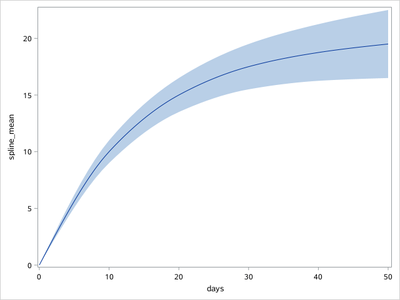## Obtaining values resulting from smoothconnect

Hi there,

I have a few data points (a simple series) that I would like to plot using some form of interpolation, and after a good bit of exploration: proc sgplot, with the series statement and the smoothconnect option has been producing the best looking results by a good margin (I explored splines and loess but these do not produce what I am looking for).

A complication is that I need to similarly do this for the CI, and would much prefer to plot that as a band. The band statement, as far as I can tell, does not have a matching smoothing option.

So my inital plan was to use ods and abstract values from the plot (just do 3 separate series). However, ods is just giving me the original data points back, not any of the in between. I may not be looking at the right place, but trying to track down the documentation for smoothconnect just leads me to the option itself, no explanation of what is going on in the background if I was going to go down the route of trying to replicate the approach elsewhere to set up the input for the plot.

Is there some way I can get those values? SAS must have them in the background somewhere, right?

Thank you

1 ACCEPTED SOLUTION

Accepted SolutionsKsharp
Super User

## Re: Obtaining values resulting from smoothconnect

``````/*
You could try  function msplint() to get spline.
*/
data thedata;
input days main lower upper;
datalines;
0 0 0 0
10 10 9 11
20 15 13.5 16.5
30 17.5 15.5 19.5
40  18.75 16.25 21.25
50 19.5 16.5 22.5
;
run;

proc sql noprint;
select count(*) into :nobs from thedata;
select min(days),max(days) into :x_min, :x_max from thedata;
select days into :x separated by ',' from thedata;
select main into :mean separated by ',' from thedata;
select lower into :lower separated by ',' from thedata;
select upper into :upper separated by ',' from thedata;
quit;

data msplint;
do days=&x_min. to &x_max. by 1;
spline_mean=msplint(days, &nobs.,&x., &mean.);
spline_lower=msplint(days, &nobs.,&x., &lower.);
spline_upper=msplint(days, &nobs.,&x., &upper.);
output;
end;
run;

proc sgplot data=msplint noautolegend;
band x=days lower=spline_lower upper=spline_upper;
series x=days y=spline_mean / smoothconnect;
run;``````5 REPLIES 5

## Re: Obtaining values resulting from smoothconnect

If you use a series plot statement along with the band plot and use the bandplot MODELNAME option to point to the series plot it should use the same interpolation.

Without data to test or even example code you have been using I have to make some guesses (Hint)

Basically something similar to:

```proc sgplot data=have;
band x=xvar y=yvar upper=ucl lower=lcl / modelplotname='myplot' ;
series x=xvar y=yvar / name='myplot' smoothconnect;
run;```

## Re: Obtaining values resulting from smoothconnect

Thank you. I include an example dataset that is roughly in the spirit of my data, and the plot code.

``````data thedata;
input days main lower upper;
datalines;
0 0 0 0
10 10 9 11
20 15 13.5 16.5
30 17.5 15.5 19.5
40  18.75 16.25 21.25
50 19.5 16.5 22.5
;
run;

proc sgplot data=thedata;
ods output sgplot=sg;
band x=days lower=lower upper=upper;
series x=days y=main / smoothconnect;
run;**per the suggestion;proc sgplot data=thedata;ods output sgplot=sg;
band x=days lower=lower upper=upper / modelname="myplot" ;
series x=days y=main / name="myplot" smoothconnect;
run;``````

In my attempt at applying the modelname to the band it looked similar to the version without it.

## Re: Obtaining values resulting from smoothconnect

A problem with the idea of running three "smoothconnect" curves (lower, upper, main) is that the interpolation of the upper/lower values is not the same as the band for the model that created them.

Can you say more about how you obtained the main, lower, and upper values? What procedure did you use?  If the main/lower/upper variables are the result of a statistical model, then the best method is to return to the procedure that created the model. You need to score the model on a dense grid of days, such as days=1 to 50 by 1.  That will give you predicted values and CIs for the model, which you can then plot.  I can provide SAS code if you post the model and procedure that you are using.Ksharp
Super User

## Re: Obtaining values resulting from smoothconnect

``````/*
You could try  function msplint() to get spline.
*/
data thedata;
input days main lower upper;
datalines;
0 0 0 0
10 10 9 11
20 15 13.5 16.5
30 17.5 15.5 19.5
40  18.75 16.25 21.25
50 19.5 16.5 22.5
;
run;

proc sql noprint;
select count(*) into :nobs from thedata;
select min(days),max(days) into :x_min, :x_max from thedata;
select days into :x separated by ',' from thedata;
select main into :mean separated by ',' from thedata;
select lower into :lower separated by ',' from thedata;
select upper into :upper separated by ',' from thedata;
quit;

data msplint;
do days=&x_min. to &x_max. by 1;
spline_mean=msplint(days, &nobs.,&x., &mean.);
spline_lower=msplint(days, &nobs.,&x., &lower.);
spline_upper=msplint(days, &nobs.,&x., &upper.);
output;
end;
run;

proc sgplot data=msplint noautolegend;
band x=days lower=spline_lower upper=spline_upper;
series x=days y=spline_mean / smoothconnect;
run;``````## Re: Obtaining values resulting from smoothconnect

Thank you! It appears that this is what smoothconnect is actually doing in the background (when I overlayed this approach and the smoothconnect the plot looked the same).

Discussion stats
• 5 replies
• 357 views
• 2 likes
• 4 in conversation